#### Local formulation

The concept of isotropic damage is appropriate for materials weakened by voids, but if the physical source of damage is the initiation and propagation of microcracks, isotropic stiffness degradation can be considered only as a first rough approximation. More refined damage models take into account the highly oriented nature of cracking, which is reflected by the anisotropic character of the damaged stiffness or compliance matrices.

A number of anisotropic damage formulations have been proposed in the literature. Here we use a model outlined by Jirásek , which is based on the principle of energy equivalence and on the construction of the inverse integrity tensor by integration of a scalar over all spatial directions. Since the model uses certain concepts from the microplane theory, it is called the microplane-based damage model (MDM).

The general structure of the MDM model is schematically shown in Fig. 7 and the basic equations are summarized in Tab. 26. Here,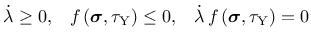and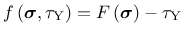are the (nominal) second-order strain and stress tensors with componentsand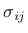;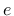and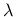are first-order strain and stress tensors with components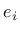and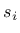, which characterize the strain and stress on microplanes'' of different orientations given by a unit vector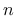with components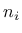;is a dimensionless compliance parameter that is a scalar but can have different values for different directions; the symboldenotes a virtual quantity; and a sumperimposed tilde denotes an effective quantity, which is supposed to characterize the state of the intact material between defects such as microcracks or voids.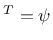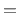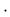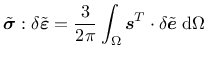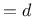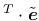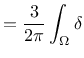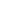d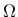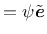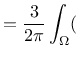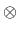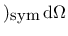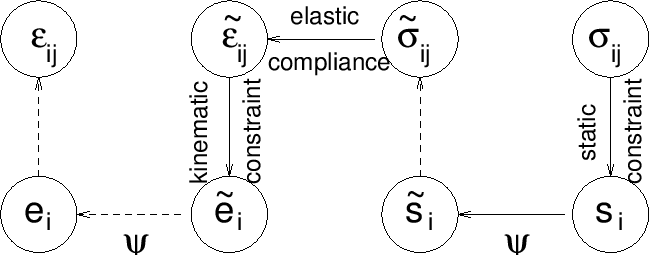Combining the basic equations, it is possible to show that the components of the damaged material compliance tensor are given by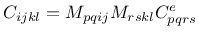(80)

where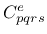are the components of the elastic material compliance tensor,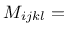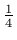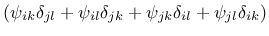(81)

are the components of the so-called damage effect tensor, and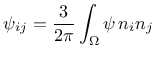d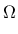(82)

are the components of the second-order inverse integrity tensor. The integration domainis the unit hemisphere. In practice, the integral over the unit hemisphere is evaluated by summing the contribution from a finite number of directions, according to one of the numerical integration schemes that are used by microplane models.

The scalar variablecharacterizes the relative compliance in the direction given by the vector. Ifis the same in all directions, the inverse integrity tensor evaluated from (82) is equal to the unit second-order tensor (Kronecker delta) multiplied by, the damage effect tensor evaluated from (81) is equal to the symmetric fourth-order unit tensor multiplied by, and the damaged material compliance tensor evaluated from (80) is the elastic compliance tensor multiplied by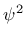. The factor multiplying the elastic compliance tensor in the isotropic damage model is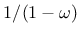, and socorresponds to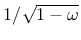. In the initial undamaged state,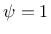in all directions. The evolution ofis governed by the history of the projected strain components. In the simplest case,is driven by the normal strain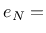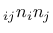. Analogy with the isotropic damage model leads to the damage law(83)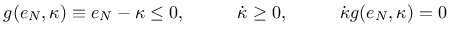(84)

in which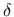is a history variable that represents the maximum level of normal strain in the given direction ever reached in the previous history of the material. An appropriate modification of the exponential softening law leads to the damage law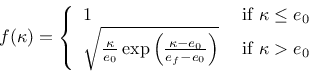(85)

where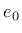is a parameter controlling the elastic limit, and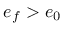is another parameter controlling ductility. Note that softening in a limited number of directions does not necessarily lead to softening on the macroscopic level, because the response in the other directions remains elastic. Therefore,corresponds to the elastic limit but not to the state at peak stress.

If the MDM model is used in its basic form described above, the compressive strength turns out to depend on the Poisson ratio and, in applications to concrete, its value is too low compared to the tensile strength. The model is designed primarily for tensile-dominated failure, so the low compressive strength is not considered as a major drawback. Still, it is desirable to introduce a modification that would prevent spurious compressive failure in problems where moderate compressive stresses appear. The desired effect is achieved by redefining the projected strain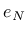as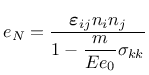(86)

where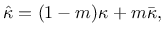is a nonnegative parameter that controls the sensitivity to the mean stress,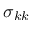is the trace of the stress tensor, and the normalizing factor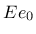is introduced in order to render the parameterdimensionless. Under compressive stress states (characterized by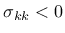), the denominator in (86) is larger than 1, and the projected strain is reduced, which also leads to a reduction of damage. A typical recommended value of parameteris 0.05.

Borek Patzak
2018-01-02